## Free Statistics

of Irreproducible Research!

Author's title
Author*Unverified author*
R Software Modulerwasp_sdplot.wasp
Title produced by softwareStandard Deviation Plot
Date of computationThu, 27 Dec 2012 16:20:17 -0500
Cite this page as followsStatistical Computations at FreeStatistics.org, Office for Research Development and Education, URL https://freestatistics.org/blog/index.php?v=date/2012/Dec/27/t1356643242ztb0r68aby65c89.htm/, Retrieved Tue, 31 Jan 2023 06:46:13 +0000
Statistical Computations at FreeStatistics.org, Office for Research Development and Education, URL https://freestatistics.org/blog/index.php?pk=204795, Retrieved Tue, 31 Jan 2023 06:46:13 +0000
QR Codes:Original text written by user:
IsPrivate?No (this computation is public)
User-defined keywords
Estimated Impact94
Family? (F = Feedback message, R = changed R code, M = changed R Module, P = changed Parameters, D = changed Data)
-     [(Partial) Autocorrelation Function] [Autocorrelatie In...] [2012-11-12 10:59:56] [41982c7b3984978a38ca838fef047984]
- RMPD  [Bootstrap Plot - Central Tendency] [Bootstrap Plot (m...] [2012-12-27 20:12:14] [41982c7b3984978a38ca838fef047984]
- R P     [Bootstrap Plot - Central Tendency] [Bootstrap Plot (m...] [2012-12-27 20:14:03] [41982c7b3984978a38ca838fef047984]
- RMPD      [Blocked Bootstrap Plot - Central Tendency] [Bootstrap Plot (g...] [2012-12-27 20:38:33] [41982c7b3984978a38ca838fef047984]
- R  D        [Blocked Bootstrap Plot - Central Tendency] [Bootstrap Plot (g...] [2012-12-27 20:42:04] [41982c7b3984978a38ca838fef047984]
- RM              [Standard Deviation Plot] [Spreidingsgrafiek...] [2012-12-27 21:20:17] [97ff841fcf87514e420f2e9629cfd808] [Current]
Feedback Forum

Post a new message
Dataseries X:
0.75
0.75
0.77
0.78
0.79
1.01
1.16
1.14
1.12
1.1
1.1
1.1
1.1
1.09
1.09
1.1
1.1
1.17
1.15
1.04
0.94
0.88
0.85
0.85
0.85
0.84
0.83
0.8
0.78
1.02
1.19
1.1
0.96
0.87
0.83
0.82
0.81
0.78
0.79
0.8
0.79
0.97
1.01
0.92
0.87
0.84
0.81
0.81
0.83
0.83
0.85
0.88
0.89
1.21
1.32
1.33
1.23
1.16
1.12
1.06
1.08
1.09
1.03
1.04
1.05
1.19
1.14
1.05
0.95
0.87
0.86
0.85

 Summary of computational transaction Raw Input view raw input (R code) Raw Output view raw output of R engine Computing time 5 seconds R Server 'Sir Maurice George Kendall' @ kendall.wessa.net

\begin{tabular}{lllllllll}
\hline
Summary of computational transaction \tabularnewline
Raw Input & view raw input (R code)  \tabularnewline
Raw Output & view raw output of R engine  \tabularnewline
Computing time & 5 seconds \tabularnewline
R Server & 'Sir Maurice George Kendall' @ kendall.wessa.net \tabularnewline
\hline
\end{tabular}
%Source: https://freestatistics.org/blog/index.php?pk=204795&T=0

[TABLE]
[ROW][C]Summary of computational transaction[/C][/ROW]
[ROW][C]Raw Input[/C][C]view raw input (R code) [/C][/ROW]
[ROW][C]Raw Output[/C][C]view raw output of R engine [/C][/ROW]
[ROW][C]Computing time[/C][C]5 seconds[/C][/ROW]
[ROW][C]R Server[/C][C]'Sir Maurice George Kendall' @ kendall.wessa.net[/C][/ROW]
[/TABLE]
Source: https://freestatistics.org/blog/index.php?pk=204795&T=0

Globally Unique Identifier (entire table): ba.freestatistics.org/blog/index.php?pk=204795&T=0

As an alternative you can also use a QR Code:

The GUIDs for individual cells are displayed in the table below:

 Summary of computational transaction Raw Input view raw input (R code) Raw Output view raw output of R engine Computing time 5 seconds R Server 'Sir Maurice George Kendall' @ kendall.wessa.net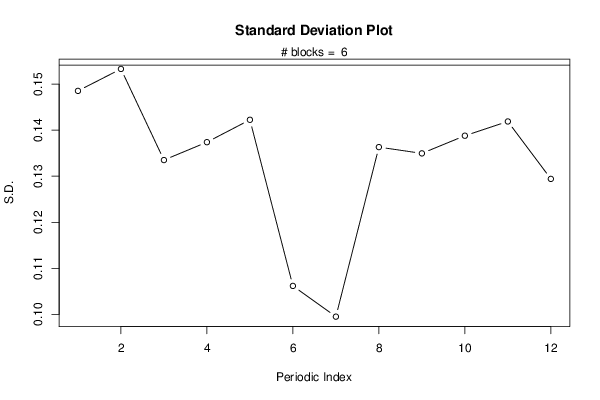PNG link Postscript link PDF link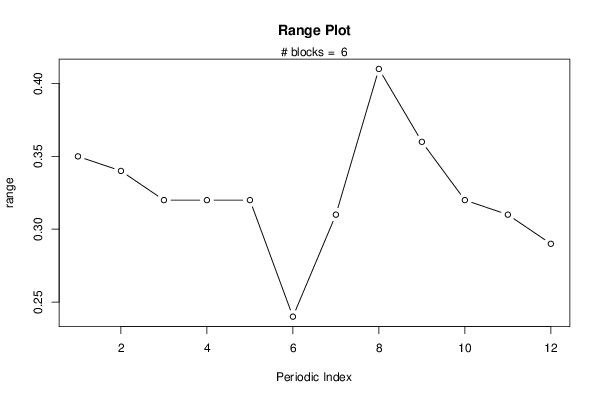PNG link Postscript link PDF link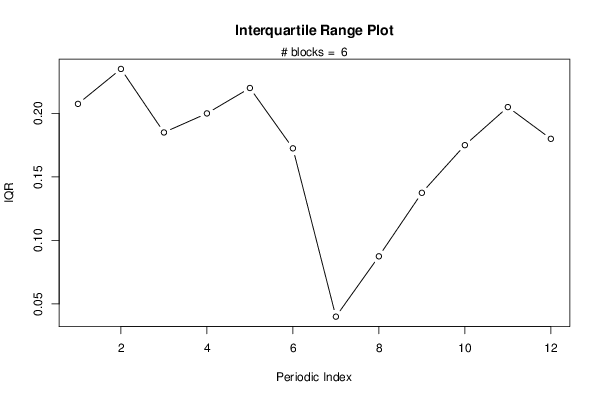PNG link Postscript link PDF link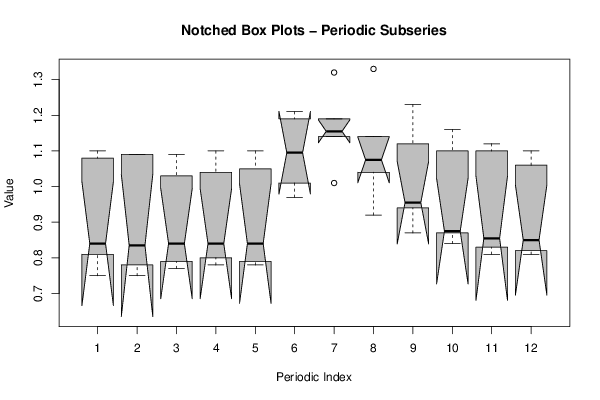PNG link Postscript link PDF link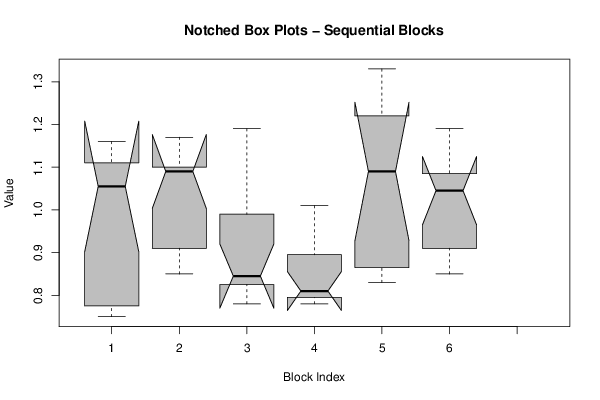PNG link Postscript link PDF link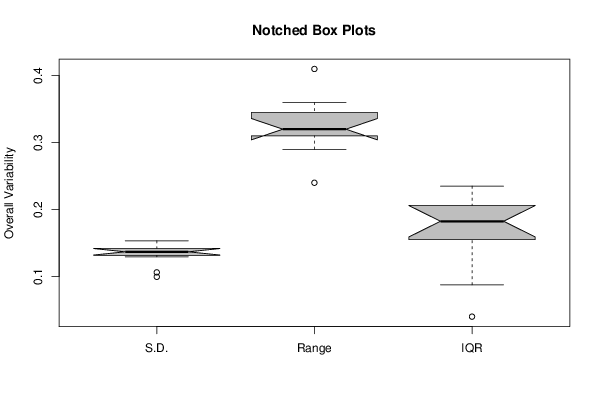PNG link Postscript link PDF link

Parameters (Session):
par1 = 50 ; par2 = 12 ;
Parameters (R input):
par1 = 12 ;
R code (references can be found in the software module):
par1 <- as.numeric(par1)(n <- length(x))(np <- floor(n / par1))arr <- array(NA,dim=c(par1,np+1))ari <- array(0,dim=par1)j <- 0for (i in 1:n){j = j + 1ari[j] = ari[j] + 1arr[j,ari[j]] <- x[i]if (j == par1) j = 0}ariarrarr.sd <- array(NA,dim=par1)arr.range <- array(NA,dim=par1)arr.iqr <- array(NA,dim=par1)for (j in 1:par1){arr.sd[j] <- sqrt(var(arr[j,],na.rm=TRUE))arr.range[j] <- max(arr[j,],na.rm=TRUE) - min(arr[j,],na.rm=TRUE)arr.iqr[j] <- quantile(arr[j,],0.75,na.rm=TRUE) - quantile(arr[j,],0.25,na.rm=TRUE)}overall.sd <- sqrt(var(x))overall.range <- max(x) - min(x)overall.iqr <- quantile(x,0.75) - quantile(x,0.25)bitmap(file='plot1.png')plot(arr.sd,type='b',ylab='S.D.',main='Standard Deviation Plot',xlab='Periodic Index')mtext(paste('# blocks = ',np))abline(overall.sd,0)dev.off()bitmap(file='plot2.png')plot(arr.range,type='b',ylab='range',main='Range Plot',xlab='Periodic Index')mtext(paste('# blocks = ',np))abline(overall.range,0)dev.off()bitmap(file='plot3.png')plot(arr.iqr,type='b',ylab='IQR',main='Interquartile Range Plot',xlab='Periodic Index')mtext(paste('# blocks = ',np))abline(overall.iqr,0)dev.off()bitmap(file='plot4.png')z <- data.frame(t(arr))names(z) <- c(1:par1)(boxplot(z,notch=TRUE,col='grey',xlab='Periodic Index',ylab='Value',main='Notched Box Plots - Periodic Subseries'))dev.off()bitmap(file='plot5.png')z <- data.frame(arr)names(z) <- c(1:np)(boxplot(z,notch=TRUE,col='grey',xlab='Block Index',ylab='Value',main='Notched Box Plots - Sequential Blocks'))dev.off()bitmap(file='plot6.png')z <- data.frame(cbind(arr.sd,arr.range,arr.iqr))names(z) <- list('S.D.','Range','IQR')(boxplot(z,notch=TRUE,col='grey',ylab='Overall Variability',main='Notched Box Plots'))dev.off()